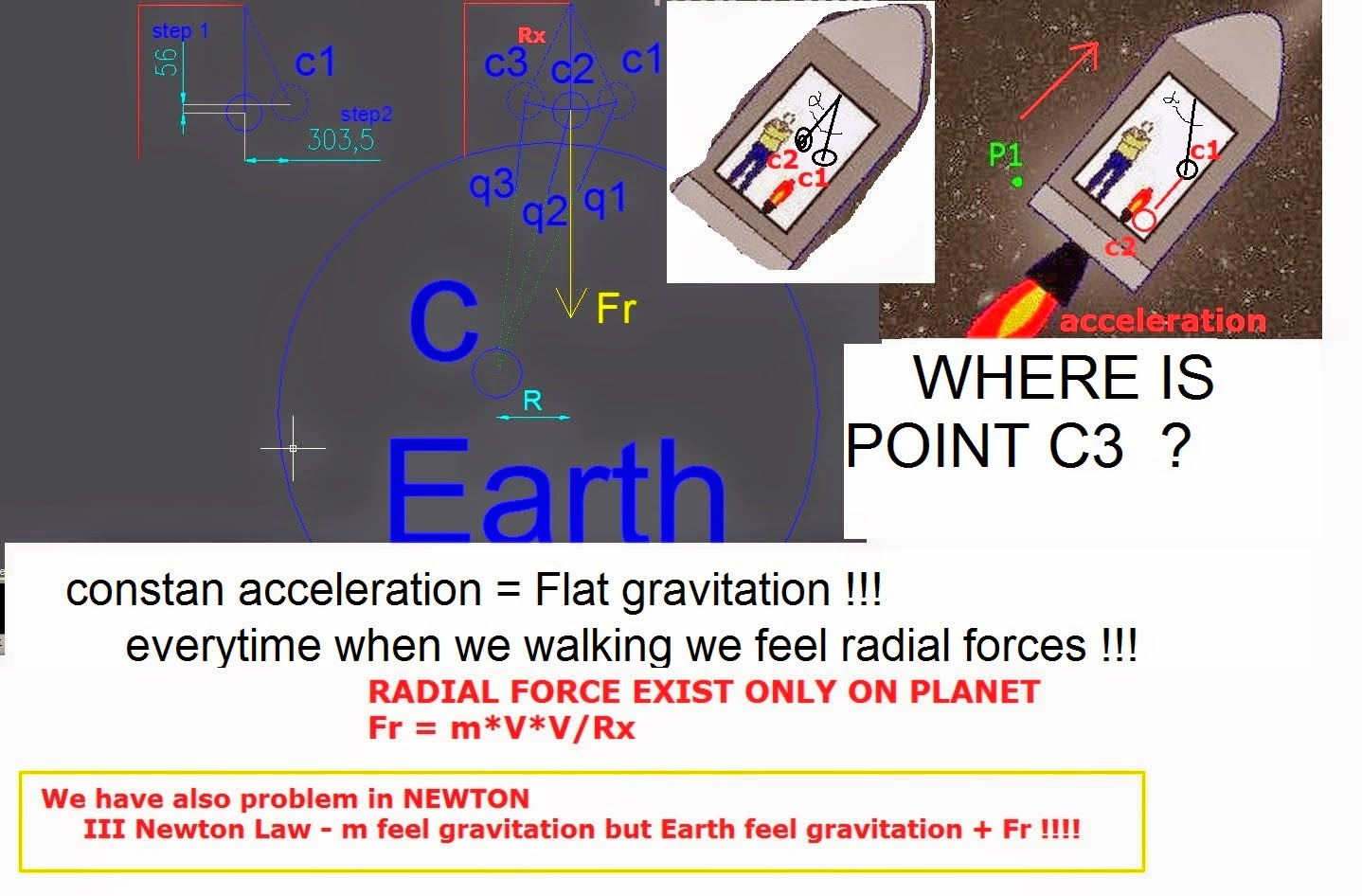NTNUJAVA Virtual Physics LaboratoryEnjoy the fun of physics with simulations! Backup site http://enjoy.phy.ntnu.edu.tw/ntnujava/June 21, 2019, 04:57:59 amWelcome, Guest. Please login or register.Did you miss your activation email? 1 Hour 1 Day 1 Week 1 Month Forever Login with username, password and session length"Talk and eat less, think and listen more." ...Wisdom
 Pages: 1    Go DownAuthor Topic: Pendulum  (Read 452558 times) 0 Members and 1 Guest are viewing this topic. Clickto toggle author information(expand message area).
Fu-Kwun Hwang
Hero MemberOffline

Posts: 3082« Embed this message Reply #30 on: June 05, 2010, 11:33:39 pm » posted from:Taipei,T\'ai-pei,TaiwanThe source code is available as attached file under the first message (Please read the topic message carefully and you should have found it).Logged
TaraLaster
NewbieOffline

Posts: 8« Embed this message Reply #31 on: December 18, 2013, 10:50:39 am » posted from:Makati,Manila,Philippines<center><applet code="pendulumSystem.class" width=500 height=350 codebase="/java/Pendulum/"><param name="Reset" value="Reset"><param name="Pause" value="Pause"><param name="Show" value="Show"><param name="Resume" value="Resume"><param name="" value=""></applet></center>

You are welcomed to check out Force analysis of a pendulum
<hr ALIGN=LEFT WIDTH="100%">
<font size=+1>How to change parameters?</font>
<ol>Set the initial position
<font color="#0000FF">Click and drag the left mouse button</font>
<ol>The horizontal position of the pendulum will follow the mouse Animation starts when you release the mouse button</ol>
<font color="#0000FF">dragging the pointer (while > holding down the left button)</font>
<ol><font color="#0000FF">from the support-point </font>(red dot) to a position that sets the length you want.</ol>
Animation starts when you release the mouse button
<li>Change gravity <font color="#FF0000">g</font></li>
<font color="#0000FF">Click near the tip of the red arrow</font>,
<ol>and drag the mouse button to change it (up-down).</ol>
<li>Change the mass of the bob</li>
<font color="#0000FF">Click near the buttom of the black stick,</font>
<ol>and drag the mouse button to change it (up-down).</ol>
</ol>
Information displayed:
<ul>1. red dots: kinetic energy <font color="#0000FF">K = m v*v /2 </font>of the bob 2. blue dots: potential energy <font color="#0000FF">U = m g h</font>of the bob
<font color="#0000FF">Try ro find out the relation between kinetic energy and pontential energy!</font> 3.black dots (pair) represent the peroid T of the pendulum
<ul>move the mouse to the dot :
<ul>will display information for that dot in the textfield</ul>
</ul></ul>
<ol>blue arrow(1): gravity green arrows(2): components of gravity red arrow
(1): velocity of the bob
<font color="#0000FF">Try to compare velocity and the tangential component of the gravitional force!</font></ol>
<hr WIDTH="100%">The calculation is in real time (use Runge-Kutta 4th order method). The period(T) is calculated when the velocity change direction.
<ul><font color="#0000FF">You can produce a period verses angle ( T - X ) curve on the screen,just started at different positions and wait for a few second.</font></ul>

Therotically, the period of a pendulum$T=\sqrt{g/L}$.
Purpose for this applet:
1. The period of the pendulum mostly depends on the length of the pendulum and the gravity (which is normally a constant)
2. The period of the pendulum is independent of the mass.
3. The variation of the pendulum due to initial angle is very small.

The equation of motion for a pendulum is$\frac{d^2\theta}{dt^2}=-\frac{g}{L}\, \sin\theta$
when the angle is small math_failure (math_unknown_error): \theta &lt;&lt; 1 ,$\sin\theta\approx \theta$
so the above equation become$\frac{d^2\theta}{dt^2}\approx-\frac{g}{L}\, \theta$
which imply it is approximately a simple harmonic motion with period$T=2\pi \sqrt{\frac{L}{g}}$

What is the error introduced in the above approximation?
From Tayler's expansion$\sin\theta=\theta-\frac{\theta^3}{3!}+\frac{\theta^5}{5!}-\frac{\theta^7}{7!}+\frac{\theta^9}{9!}-\frac{\theta^11}{11!}+...$
To get first order approximation, the error is$\frac{\theta^3}{3!}=\frac{\theta^3}{6}$
So the relative error (error in percentage)=$\frac{\theta^3/6}{\theta}=\frac{\theta^2}{6}$
If the angle is 5 degree, which mean$\theta=5*pi/180\approx=5/60=1/12$
So the relative error is$\frac{\theta^2}{6}=1/(12^2*6)=1/(144*6)=1/864\approx 0.00116$

For angle=5 degree , the relative error is less than$0.116%$
For angle=10 degree , the relative error is less than$0.463%$
For angle=20 degree , the relative error is less than$1.85%$

So the period of the pendulum is almost independent of the initial angle (the error is relatively small unless the angle is much larger than 20 degree- for more than 2% error).

So sad.. still can't find resource code.Do you have any other way?Logged

“Hard-earned good money is hard to waste; but can still have some good moments.”
― Mymathdone.com
maciejmarosz
NewbieOffline

Posts: -15« Embed this message Reply #32 on: April 18, 2014, 05:29:27 pm » posted from:Warsaw,Mazowieckie,PolandEN > http://youtu.be/-qLIjbjB0GU

PL > http://youtu.be/lmKccRTQgy4

EN > http://youtu.be/XWYFIUEaOmc  PL >http://youtu.be/rs8d1zBHgrw

http://2.bp.blogspot.com/-fKJMF7z908A/U0t2KnjvpxI/AAAAAAAABt0/ZhpYBUNw83M/s1600/ff.jpg

FIRST ENGINE THAT I MADE :

PL > http://youtu.be/YI2Vqf9TFi4
EN > http://youtu.be/iTQweoVZspc

more

http://3.bp.blogspot.com/-NsHHVMzdvzg/Uz7plOHAFTI/AAAAAAAABno/QCS96DgHd94/s1600/exp.JPG

http://1.bp.blogspot.com/-JpeYrYct79g/Uz-1Zw70DrI/AAAAAAAABn8/VGpmzWH9i3Y/s1600/mt1.jpg

EN > http://youtu.be/Aazwjy3n-fg

PL > http://youtu.be/uk9R7EylmQU

http://marosz-physics.blogspot.com/Logged
diinxcom
watchlist
Jr. MemberOffline

Posts: 25Designer« Embed this message Reply #33 on: December 14, 2014, 05:51:29 pm » posted from:,,Satellite Provider-*-
Mark it first..
I am new here.. I hope can get many thinks from this forum...Logged
 Pages: 1    Go Up
"Talk and eat less, think and listen more." ...Wisdom
 Related Topics Subject Started by Replies Views Last postPendulum in 3D Dynamics Fu-Kwun Hwang 6 26253November 19, 2015, 02:19:33 pm by lookangPendulum in 3D dynamics ahmedelshfie 1 4437June 08, 2010, 07:25:03 pm by ahmedelshfiePendulum Examples for beginner who want to learn EJS Fu-Kwun Hwang 1 5409January 05, 2019, 02:23:23 pm by vivoziPendulum dynamics ahmedelshfie 1 3840September 24, 2010, 06:58:52 pm by ahmedelshfie3D rotational pendulum also known as Furuta's pendulum by Francisco Esquembre Dynamics lookang 7 16576May 17, 2019, 11:41:14 am by angelinajolie
Page created in 1.036 seconds with 23 queries.since 2011/06/15